#0

## MS SQL 查詢的方法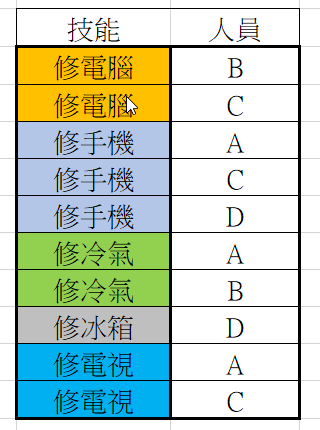### 4 個回答

1

``````IF OBJECT_ID('test.dbo.skill') IS NULL CREATE TABLE [test].[dbo].[skill]
( [skill] NVARCHAR(10) NOT NULL,
[member] NVARCHAR(10) NOT NULL);
``````

``````INSERT INTO [test].[dbo].[skill] ([skill], [member]) VALUES
(N'修電腦', 'B'), (N'修電腦', 'C'), (N'修手機', 'A'),
(N'修手機', 'C'), (N'修手機', 'D'), (N'修冷氣', 'A'),
(N'修冷氣', 'B'), (N'修冰箱', 'D'), (N'修電視', 'A'),
(N'修電視', 'C');
``````

``````SELECT COUNT(1) AS num, [member] FROM [test].[dbo].[skill]
WHERE [skill] IN ('修手機', '修冰箱')
GROUP BY [member]
HAVING COUNT(1) >= 2;
``````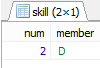1

2

``````select 人員, count(*) skillcnt
from (
-- 選二項技能
SELECT 技能, 人員
FROM TableA
where 技能='修電腦'
union
SELECT 技能, 人員
FROM TableA
where 技能='修手機'
....
...
..
) AAA
group by 人員
having count(*) = 2
``````
2
``````SELECT 人員,
STUFF((SELECT '. '+技能
FROM 人員TABLE AS B
WHERE B.人員=A.人員
FOR XML PATH(''),Type).value('.','NVARCHAR(MAX)'),1,2,'') AS 他會的技能
FROM 人員TABLE AS A
WHERE 技能 IN ('修電腦','修冰箱')
GROUP BY 人員
``````

RESULTS.......一人一串，列出會修手機或修冰箱的，用in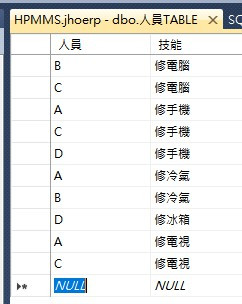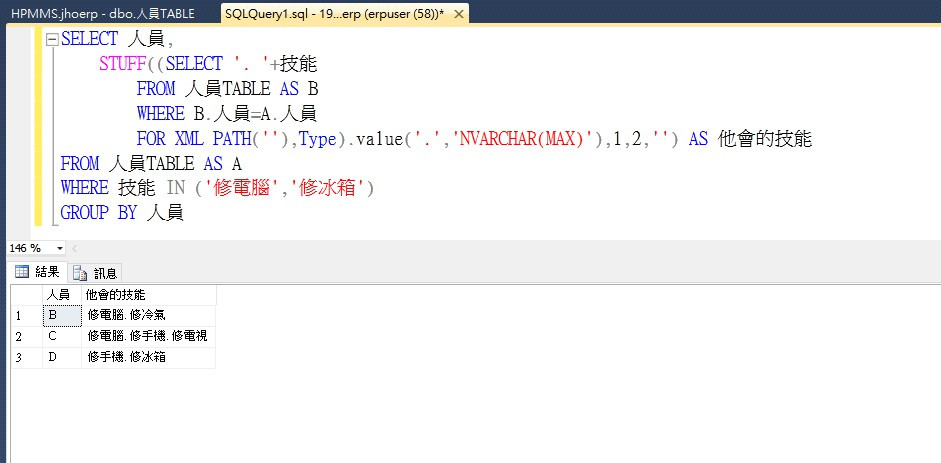'修冰箱','修電腦' 不行哦. 呵..

..

``````CREATE VIEW [dbo].[vw_技能表]
as
SELECT 人員,
STUFF((SELECT '. '+技能
FROM 人員TABLE AS B
WHERE B.人員=A.人員
FOR XML PATH(''),Type).value('.','NVARCHAR(MAX)'),1,2,'') AS 他會的技能
FROM 人員TABLE AS A
GROUP BY 人員

GO
``````

``````select * from vw_技能表
where 他會的技能 like '%修手機%' and  他會的技能 like '%修冰箱%'
``````

fuzzylee1688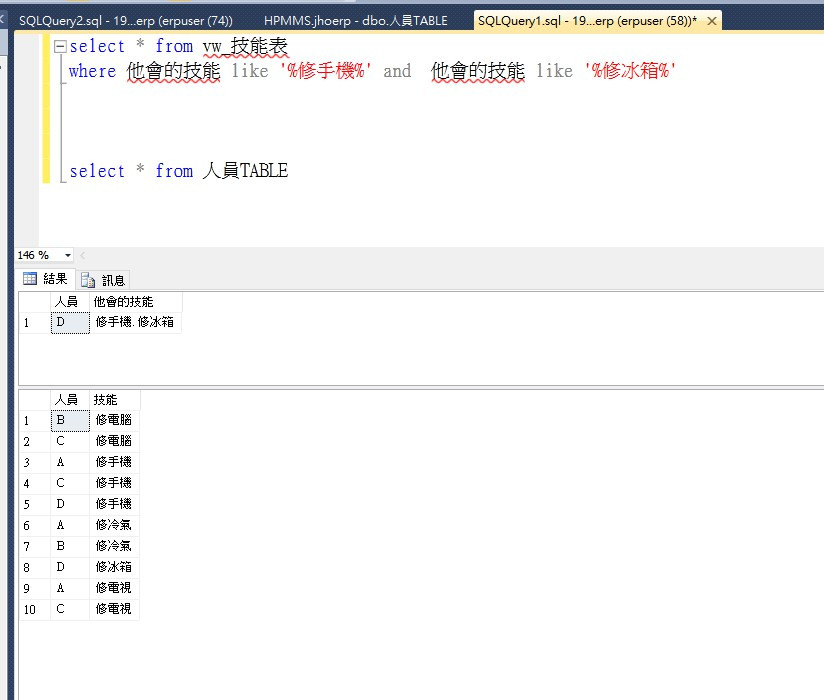``````CREATE VIEW [dbo].[vw_技能表]
as
SELECT 人員
,(SELECT 技能
FROM TABLE_NAME AS A
WHERE A.欄位1 = B.欄位1
FOR XML PATH()
)AS 他會的技能
FROM TABLE_NAME AS B
GROUP BY 人員
GO

SELECT * FROM vw_技能表 WHERE 他會的技能 = '修電腦修冰箱'
``````